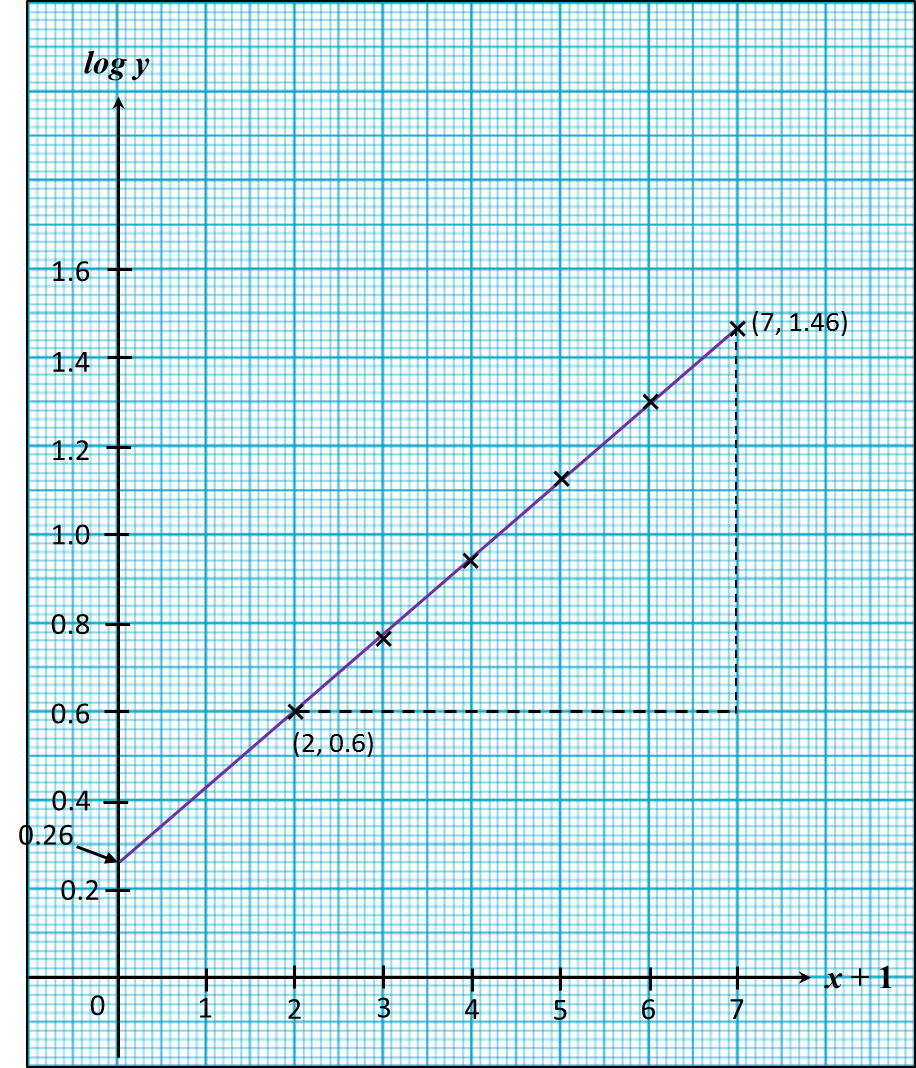\

# 6.6.5 SPM practice 3 (Linear Law) – Question 5

Question 5:
Use graph paper to answer this question.
Table 1 shows the values of two variables, x and y, obtained from an experiment. The variables x and y are related by the equation y = hkx + 1, where h and k are constants.

 x 1 2 3 4 5 6 y 4 5.7 8.7 13.2 20 28.8
Table 1

(a) Based on table 1, construct a table for the values (x + 1) and log y. [2 marks]
(b) Plot log y against (x + 1), using a scale of 2 cm to 1 uint on the (x + 1) –axis and 2 cm to 0.2 unit on the log y-axis.
Hence, draw the line of best fit.    [3 marks]
(c) Use your graph in 9(b) to find the value of
(i) h.
(ii) k.  [5 marks]

Solution:
(a)
 x + 1 2 3 4 5 6 7 log y 0.6 0.76 0.94 1.12 1.3 1.46

(b)(c)
= hkx + 1
log y = log h + (x + 1) log k
log y = log k (x + 1) + log h
Y = log y
m = log k
X = (x + 1)
c = log h

(i)
log h = y-intercept
log h = 0.26
h = 1.82

(ii)
$\begin{array}{l}\mathrm{log}k=\text{Gradient of the graph}\\ \text{}=\frac{1.46-0.60}{7-2}\\ \text{}=0.172\\ k=1.486\end{array}$

### 1 thought on “6.6.5 SPM practice 3 (Linear Law) – Question 5”

1.perkongsian bijak, m
berguna untuk saya